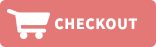Home » Shelf Strips » 1.22" X .010" PVC SHELF STRIPS (RED OR BLUE)Product ID: ShelfStrip,47.75in-red/blue100
Shelf Strips

The shelf strip measures 1.22" x 47.75" x .010".  Prices decrease as volume increases.  Store will automatically calculate discounts.100 strips per bundle, for quantities of 10 bundles or more see the 1,000 count section.

Quantity Pricing per 100:
1= \$30.89
2= \$22.72
3= \$19.80
5= \$17.64
Price: \$30.89Product ID: ShelfStrip,47.75in-red/blue1000
Shelf Strips

The shelf strip measures 1.22" x 47.75" x .010".  Prices decrease as volume increases.  Store will automatically calculate discounts.1,000 strips per case, for quantities of 900 strips or less, please see the 100 count section.

Quantity Pricing per 1,000:
1= \$150.70
2= \$145.20
3= \$136.30
5= \$126.20
10= \$118.20
25= \$109.00
50= \$103.10
100= \$96.50
Price: \$150.70Product ID: ShelfStrip,24in,red/blue100
Shelf Strips

The shelf strip measures 1.22" x 24" x .010".  Prices decrease as volume increases.  Store will automatically calculate discounts.100 strips per bundle, for quantities of 10 bundles or more see the 1,000 count section.

Quantity Pricing per 100:
1= \$21.43
2= \$15.76
3= \$14.57
5= \$12.36
Price: \$21.43Product ID: ShelfStrip,24in,red/blue1000
Shelf Strips

The shelf strip measures 1.22" x 24" x .010".  Prices decrease as volume increases.  Store will automatically calculate discounts.1,000 strips per case, for quantities of 900 strips or less, please see the 100 count section.

Quantity Pricing per 1,000:
1= \$106.5
2= \$103.8
3= \$97.4
5= \$91.10
10= \$85.20
25= \$78.70
50= \$75.10
100= \$71.60